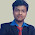#include<stdio.h>
#include<stdlib.h>

struct node
{
int coef,expo;
struct node* next;
};

struct node* insertpoly(struct node* thead,int c,int e);
struct node* append(struct node* thead,int c,int e);

void main()
{
int a,b,n,i;

// Inputing the first polynomial..

printf("Enter the no of terms of polynomial 1..");
scanf("%d",&n);
printf("\nEnter the polynomial..");
for(i=0;i<n;i++){
printf("\nEnter the coefficient and exponent of the term..");
scanf("%d%d",&a,&b);
}

// Inputing the second polynomial..

printf("\nEnter the no of terms of polynomial 2..");
scanf("%d",&n);
printf("\nEnter the polynomial..");
for(i=0;i<n;i++){
printf("\nEnter the coefficient and exponent of the term..");
scanf("%d%d",&a,&b);
}

//Displaying the polynomial..

printf("\nThe polynomial 1 is..");
printf("\nThe polynomial 2 is..");
printf("\nThe sum of the two polynomials is..");
}

struct node* append(struct node* thead,int c,int e)
{
struct node* newnode = (struct node*)malloc(sizeof(struct node));
newnode->coef=c;
newnode->expo=e;
if(thead==NULL){// Corner Case to handle if the list is empty...
newnode->next=NULL;
return newnode;
}
while(trav->next!=NULL) // Traversing to point to the last node...
trav=trav->next;
trav->next=newnode;
newnode->next=NULL;
}

struct node* insertpoly(struct node* thead,int c,int e)
{
struct node* newnode=(struct node*)malloc(sizeof(struct node));
newnode->coef=c;
newnode->expo=e;
if(thead==NULL){            // for inserting the first node..
newnode->next=NULL;
return newnode;
}
struct node* prev,* curr;
while(curr!=NULL && curr->expo>e){
prev=curr;
curr=curr->next;
}
if(curr==thead){            // for inserting before the first node...
newnode->next=curr;
return newnode;
}
else if(curr==NULL){        //for inserting after the last node....
prev->next=newnode;
newnode->next=NULL;
}
else{
newnode->next=curr;
prev->next=newnode;
}
}

{
struct node* ans=NULL;
struct node* t1,* t2;
while(t1!=NULL && t2!=NULL){
if(t1->expo > t2->expo){
ans=append(ans,t1->coef,t1->expo);
t1=t1->next;
}
else if(t1->expo < t2->expo){
ans=append(ans,t2->coef,t2->expo);
t2=t2->next;
}
else{
ans=append(ans,(t1->coef)+(t2->coef),t1->expo);
t1=t1->next;
t2=t2->next;
}
}

while(t1!=NULL){            //coping the remaining terms of polynomial 1...
ans=append(ans,t1->coef,t1->expo);
t1=t1->next;
}

while(t2!=NULL){            //coping the remaining terms of polynomial 2...
ans=append(ans,t2->coef,t2->expo);
t2=t2->next;
}
return ans;
}

{
if(temp==NULL){
printf("\nEmpty..");
}
else{
while(temp->next!=NULL){
printf(" %dx^%d +",temp->coef,temp->expo);
temp=temp->next;
}
printf(" %dx^%d ",temp->coef,temp->expo);
}
}

1.This program is really very good.Here there is no drawbacks.It solve all the cases (i.e) even if the user enters unsorted polynomail

2.good program!!! It cleared my doubts

3.you are the best bro

4.attach subtract and multi operations to it

5.6.please attach subtract and multu to it

7.Good explanation

8.9.10.thank you, do more of this

11.What is the output of this program?

12.13.14.15.the code is really good solving all case and conditions
well done

16.17.I need explination of this code